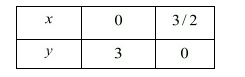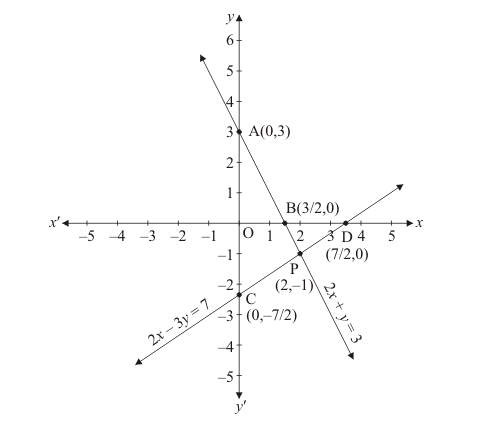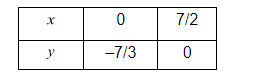# Solve the following systems of equations graphically:

Question:

Solve the following systems of equations graphically:

$2 x+y-3=0$

$2 x-3 y-7=0$

Solution:

The given equations are

$\Rightarrow 2 x+y=3$ $\ldots . .(i)$

$\Rightarrow 2 x-3 y=7$ $\ldots . .(ii)$

Putting $x=0$ in equation $(i)$, we get:

$\Rightarrow 2 \times 0+y=3$

$\Rightarrow y=3$

$x=0, \quad y=3$

Putting $y=0$ in equation $(i,$, we get:

$\Rightarrow 2 x+0=3$

$\Rightarrow x=3 / 2$

$x=3 / 2, \quad y=0$

Use the following table to draw the graph.Draw the graph by plotting the two points $A(0,3)$ and $B(3 / 2,0)$ from table.Graph of the equation....(ii):

$2 x-3 y=7$

Putting $x=0$ in equation (ii) we get:

$\Rightarrow 2 \times 0-3 y=7$

$\Rightarrow y=-7 / 3$

$x=0, \quad y=-7 / 3$

Putting $y=0$ in equation $(i i)$, we get

Use the following table to draw the graph.Draw the graph by plotting the two points $C(0,-7 / 2)$ and $D(7 / 2,0)$ from table.

The two lines intersect at points $\mathrm{P}(2,-1)$.

Hence $x=2, y=-1$ is the solution.